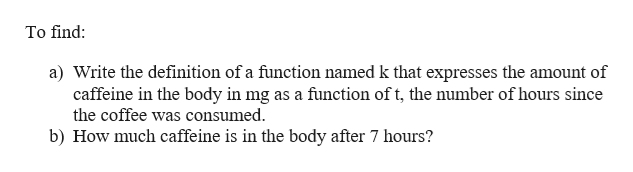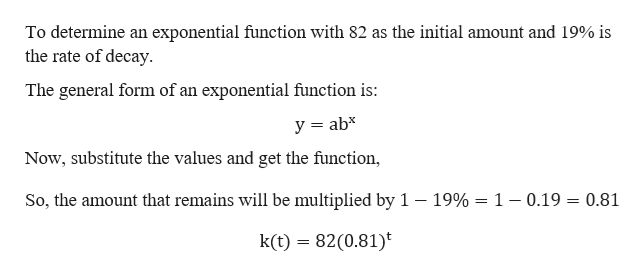# A typical cup of coffee contains about 82 mg of caffeine and every hour approximately 19% is metabolized and eliminated.Write the definition of a function named k that expresses the amount of caffeine in the body in mg as a function of t, the number of hours since the coffee was consumed. How much caffeine is in the body after 7 hours?mg A 80 mg of a vaccine is given to a patient to prevent measles.  The vaccine leaves the body at a rate of 4.8% per hour.Define a function g that gives the mass of the vaccine remaining in the body, V, in terms of the number of hours, n since the vaccine was administered.How much of the vaccine remains in the body 24 hours after it was administered?mg  Use your graphing calculator to determine how many hours passed before there were only 5mg of the vaccine remaining in the body.hours

Question
82 views

A typical cup of coffee contains about 82 mg of caffeine and every hour approximately 19% is metabolized and eliminated.

1. Write the definition of a function named k that expresses the amount of caffeine in the body in mg as a function of t, the number of hours since the coffee was consumed.

• How much caffeine is in the body after 7 hours?

mg

A 80 mg of a vaccine is given to a patient to prevent measles.  The vaccine leaves the body at a rate of 4.8% per hour.

1. Define a function g that gives the mass of the vaccine remaining in the body, V, in terms of the number of hours, n since the vaccine was administered.

How much of the vaccine remains in the body 24 hours after it was administered?

mg

• Use your graphing calculator to determine how many hours passed before there were only 5mg of the vaccine remaining in the body.

hours

check_circle

Step 1

Given that a typical cup of coffee contains about 82 mg of caffeine and every hour approximately 19% is metabolized and eliminated.help_outlineImage TranscriptioncloseTo find: a) Write the definition of a function named k that expresses the amount of caffeine in the body in mg as a function of t, the number of hours since the coffee was consumed. b) How much caffeine is in the body after 7 hours? fullscreen
Step 2

a) Initially the amount of caffeine is 82 mg. It is eliminated at a rate of 19% per hour.help_outlineImage TranscriptioncloseTo determine an exponential function with 82 as the initial amount and 19% is the rate of decay. The general form of an exponential function is: y ab Now, substitute the values and get the function, So, the amount that remains will be multiplied by 1 - 19% = 1- 0.19 = 0.81 k(t) 82(0.81) = fullscreen
Step 3

b) Now, to determine the amount of caff...

### Want to see the full answer?

See Solution

#### Want to see this answer and more?

Solutions are written by subject experts who are available 24/7. Questions are typically answered within 1 hour.*

See Solution
*Response times may vary by subject and question.
Tagged in

### Functions